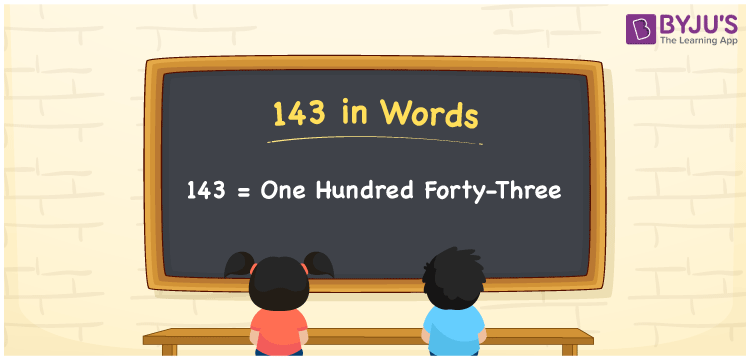# 143 in Words

143 in words is written as One hundred forty-three. In both the International System of Numerals and the Indian System of Numerals, 143 is written as One hundred forty-three. The number 143 is a Cardinal Number as it represents some quantity. For example, “there are 143 people in the room”.

 143 in Words One hundred forty-three One hundred forty-three in Number 143

## 143 in English Words

We write 143 in English Words using the letters of the English alphabet. Therefore, we read 143 in English as “One hundred forty-three.”## How to Write 143 in Words?

To write 143 in words, we shall use the place value chart. In the place value chart, write 1 in the hundreds, 4 in the tens, and 3 in the ones, respectively. Now let us make a place value chart to write the number 143 in words.

 Hundreds Tens Ones 1 4 3

Thus, we can write the expanded form as

1 × Hundred + 4 × Ten + 3 × One

= 1 × 100 + 4 × 10 + 3 × 1

= 100 + 40 + 3

= 143

= One hundred forty-three.

143 is a natural number, the successor of 142 and the predecessor of 144.

143 in words – One hundred forty-three

• Is 143 an odd number? – Yes
• Is 143 an even number? – No
• Is 143 a perfect square number? – No
• Is 143 a perfect cube number? – No
• Is 143 a prime number? – No
• Is 143 a composite number? – Yes

## Frequently Asked Questions on 143 in Words

Q1

### How to write 143 in words?

143 in words is written as One hundred forty-three.
Q2

### How to write 143 in the International and Indian System of Numerals?

In both, the system of numerals, 143 in words, is written as One hundred forty-three.
Q3

### What are the factors of 143?

The factors of 143 are 1, 11, 13 and 143.